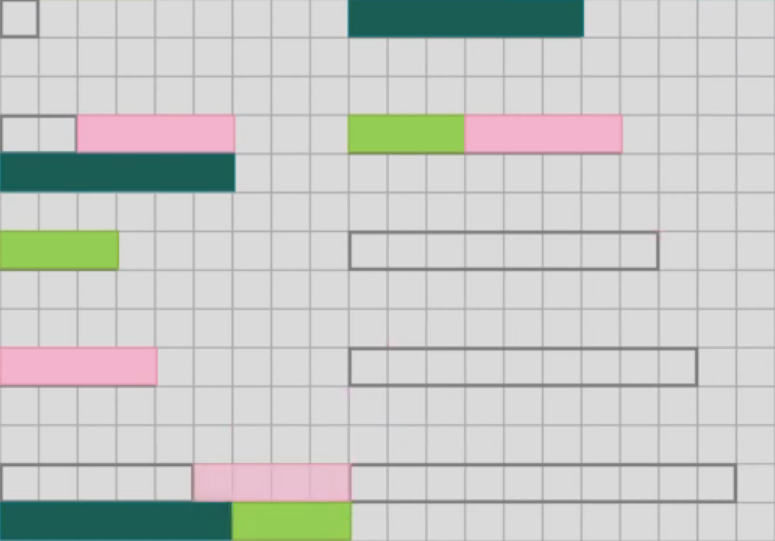#### You may also like### Pair Sums

Five numbers added together in pairs produce: 0, 2, 4, 4, 6, 8, 9, 11, 13, 15 What are the five numbers?### Summing Consecutive Numbers

15 = 7 + 8 and 10 = 1 + 2 + 3 + 4. Can you say which numbers can be expressed as the sum of two or more consecutive integers?### Big Powers

Three people chose this as a favourite problem. It is the sort of problem that needs thinking time - but once the connection is made it gives access to many similar ideas.

# Combining Lengths

### Why do this problem?

This low threshold, high ceiling task provides an excellent opportunity for students to work systematically and consider possible combinations of numbers, with the potential to discover and explain patterns involving number bases, powers and sequences.

### Possible approach

Introduce the problem with the three rods from the example: light green (3), pink (4) and dark green (6). Invite students to suggest how they could be combined to make other lengths.

They are likely to think of combining the rods by adding first of all, and may need prompting to come up with arrangements where the rods overlap to make lengths using differences, or a combination of addition and subtraction. This image might be useful to demonstrate what is allowed:Then challenge students to find sets of three rods that can be used to make all the numbers from 1-10. If Cuisenaire rods are available, students could use them. Alternatively, students could use the online Cuisenaire environment in the problem, or work on squared paper.

Once students find a set that can make all the numbers from 1-10, invite them to move on and consider the question: "What's the maximum number of different consecutive lengths that can be measured in this way with three rods?"

There are different ways that students might approach this. One useful strategy is to start by finding the maximal set with just two rods and then think about how to extend it (rods of length 1 and 3 can be used to make all the numbers from 1 to 4, so choose a new rod to make numbers from 5 onwards). Alternatively, students could think about all the ways that up to three numbers can be combined with + and -, and which combinations give positive answers.

Finally, students who have found the maximal set could be invited to explain their findings to the class, and this can be used as a stepping stone to predict which numbers should be used for making different lengths with 4, 5, 6... rods.

Key questions

Are there any numbers that you can make with your rods in more than one way? This is a waste, so how can you avoid this?
If I can make all the lengths from 1 to n with my set of rods, which rod should I add next?

Possible support

Start by considering how many different lengths can be made with just two rods.

Possible extension

Students could explore why the sum of successive powers of 3 always gives an answer of the form $\frac{3^n - 1}2$